# 1431 is a Triangular Number and a Hexagonal Number

If you only look at a list of triangular numbers or a list of hexagonal numbers, you might miss the relationship that figurate numbers have with each other.

1378 is the 52nd triangular number, and you can use it to find the 53rd triangular number (1431), the 53rd square number, the 53rd pentagonal number, and so forth.

351 is the 26th triangular number, and you can use it to find the 27th triangular number, the 27th square number, the 27th pentagonal number, the 27th hexagonal number (1431), and so forth.

See the relationship in the graphic below:Should you get excited that 1431 is BOTH a triangular number and a hexagonal number? Not really. It turns out that every hexagonal number is also a triangular number. (But not every triangular number is a hexagonal number.)

Here are a few more facts about the number 1431:

• 1431 is a composite number.
• Prime factorization: 1431 = 3 × 3 × 3 × 53, which can be written 1431 = 3³ × 53
• 1431 has at least one exponent greater than 1 in its prime factorization so √1431 can be simplified. Taking the factor pair from the factor pair table below with the largest square number factor, we get √1431 = (√9)(√159) = 3√159
• The exponents in the prime factorization are 3 and 1. Adding one to each exponent and multiplying we get (3 + 1)(1 + 1) = 4 × 2 = 8. Therefore 1431 has exactly 8 factors.
• The factors of 1431 are outlined with their factor pair partners in the graphic below.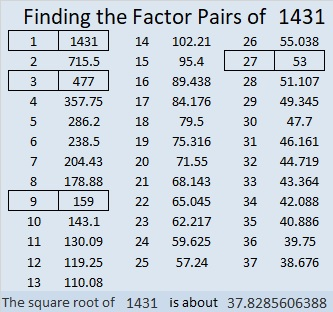1431 is the hypotenuse of a Pythagorean triple:
756-1215-1431 which is 27 times (28-45-53)

# 1430 Is a Catalan Number

1430 is the eighth Catalan number because it is equal to (2⋅8)!÷((8+1)!8!):
10 × 11 × 12 × 13 × 14 × 15 × 16 ÷( 1 × 2 × 3 × 4 × 5 × 6 × 7 × 8 ) = 1430.

For example, the vertices of a decagon can also be the vertices of eight triangles. Those eight triangles can be drawn in 1430 different ways. Here are a few of those ways: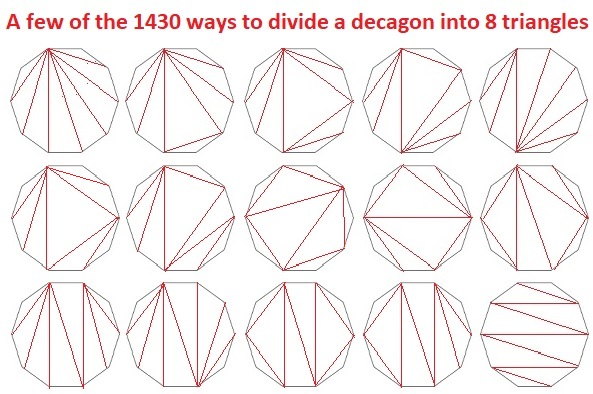Some of the 1430 ways are rotations and/or reflections of the ways illustrated above. Many of the 1430 ways are NOT represented in that graphic at all. It would be mind-boggling to draw all 1430 ways!

Here are some other facts about the number 1430:

• 1430 is a composite number.
• Prime factorization: 1430 = 2 × 5 × 11 × 13
• 1430 has no exponents greater than 1 in its prime factorization, so √1430 cannot be simplified.
• The exponents in the prime factorization are 1, 1, 1, and 1. Adding one to each exponent and multiplying we get (1 + 1)(1 + 1)(1 + 1)(1 + 1) = 2 × 2 × 2 × 2 = 16. Therefore 1430 has exactly 16 factors.
• The factors of 1430 are outlined with their factor pair partners in the graphic below.1430 is the hypotenuse of FOUR Pythagorean triples:
352-1386-1430 which is 22 times (16-63-65)
550-1320-1430 which is (5-12-13) times 110
726-1232-1430 which is 22 times (33-56-65)
858-1144-1430 which is (3-4-5) times 286

# 1429 Find the Factors Challenge

I wanted today’s puzzle to look like a big candy bar, but I don’t think I succeeded. I hope you will still think it is the best treat you got today! Good luck!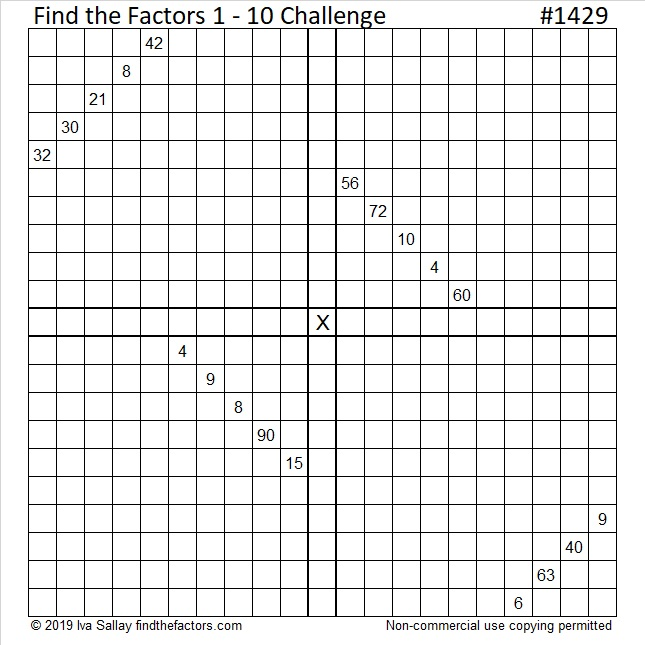Print the puzzles or type the solution in this excel file: 12 Factors 1419-1429

Now I’ll tell you some facts about the number 1429:

• 1429 is a prime number.
• Prime factorization: 1429 is prime.
• 1429 has no exponents greater than 1 in its prime factorization, so √1429 cannot be simplified.
• The exponent in the prime factorization is 1. Adding one to that exponent we get (1 + 1) = 2. Therefore 1429 has exactly 2 factors.
• The factors of 1429 are outlined with their factor pair partners in the graphic below.

How do we know that 1429 is a prime number? If 1429 were not a prime number, then it would be divisible by at least one prime number less than or equal to √1429. Since 1429 cannot be divided evenly by 2, 3, 5, 7, 11, 13, 17, 19, 23, 29 31, or 37, we know that 1429 is a prime number.Stetson.edu informs us that 1429² = 2,042,041. That’s the smallest perfect square whose first three digits are repeated in order by the next three digits.

1429 is the sum of two squares:
30² + 23² = 1429

1429 is the hypotenuse of a primitive Pythagorean triple:
371-1380-1429 calculated from 30² – 23², 2(30)(23), 30² + 23²

Here’s another way we know that 1429 is a prime number: Since its last two digits divided by 4 leave a remainder of 1, and 30² + 23² = 1429 with 30 and 23 having no common prime factors, 1429 will be prime unless it is divisible by a prime number Pythagorean triple hypotenuse less than or equal to √1429. Since 1429 is not divisible by 5, 13, 17, 29, or 37, we know that 1429 is a prime number.

# 1427 Mysterious Cat

This mysterious Halloween cat shares twelve clues that can help you solve its puzzle. Each clue is the products of two factors from 1 to 12 multiplied together. Will you be able to solve its mystery?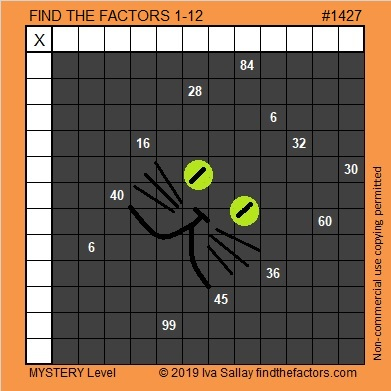Print the puzzles or type the solution in this excel file: 12 Factors 1419-1429

Now I’ll tell you a little bit about the puzzle number, 1427:

• 1427 is a prime number.
• Prime factorization: 1427 is prime.
• 1427 has no exponents greater than 1 in its prime factorization, so √1427 cannot be simplified.
• The exponent in the prime factorization is 1. Adding one to that exponent we get (1 + 1) = 2. Therefore 1427 has exactly 2 factors.
• The factors of 1427 are outlined with their factor pair partners in the graphic below.

How do we know that 1427 is a prime number? If 1427 were not a prime number, then it would be divisible by at least one prime number less than or equal to √1427. Since 1427 cannot be divided evenly by 2, 3, 5, 7, 11, 13, 17, 19, 23, 29 31, or 37, we know that 1427 is a prime number.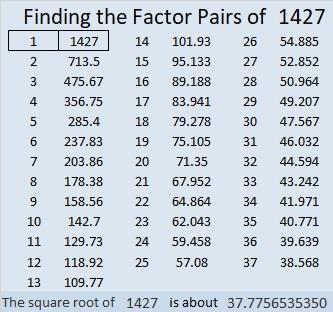1427 is 272 in BASE 25 because 2(25²) + 7(25) + 2(1) = 1427

# 1425 Jack O’Lantern

Most of my puzzles don’t have as many clues as this Jack O’lantern puzzle has. Those extra clues could make it easier to solve. On the other hand, some of the clues might still be tricky.Print the puzzles or type the solution in this excel file: 12 Factors 1419-1429

Here are some facts about the puzzle number, 1425:

• 1425 is a composite number.
• Prime factorization: 1425 = 3 × 5 × 5 × 19, which can be written 1425 = 3 × 5² × 19
• 1425 has at least one exponent greater than 1 in its prime factorization so √1425 can be simplified. Taking the factor pair from the factor pair table below with the largest square number factor, we get √1425 = (√25)(√57) = 5√57
• The exponents in the prime factorization are 1, 2, and 1. Adding one to each exponent and multiplying we get (1 + 1)(2 + 1)(1 + 1) = 2 × 3 × 2 = 12. Therefore 1425 has exactly 12 factors.
• The factors of 1425 are outlined with their factor pair partners in the graphic below.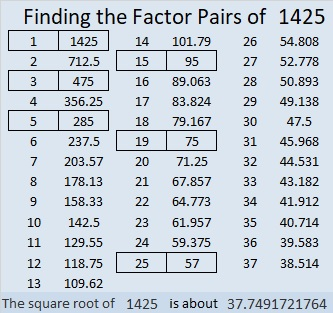1425 is the hypotenuse of TWO Pythagorean triples:
399-1368-1425 which is (7-24-25) times 57
855-1140-1425 which is (3-4-5) times 285

# 1424 Logic and Fear

Should this puzzle excite fear in you or anyone else? The logical answer is no, of course not. Logic can also help you to find the solution, so don’t be afraid, give it a try!Print the puzzles or type the solution in this excel file: 12 Factors 1419-1429

Finding the factors of 1424 shouldn’t bring fear to you either:

• 1424 is a composite number.
• Prime factorization: 1424 = 2 × 2 × 2 × 2 × 89, which can be written 1424 = 2⁴ × 89
• 1424 has at least one exponent greater than 1 in its prime factorization so √1424 can be simplified. Taking the factor pair from the factor pair table below with the largest square number factor, we get √1424 = (√16)(√89) = 4√89
• The exponents in the prime factorization are 4 and 1. Adding one to each exponent and multiplying we get (4 + 1)(1 + 1) = 5 × 2 = 10. Therefore 1424 has exactly 10 factors.
• The factors of 1424 are outlined with their factor pair partners in the graphic below.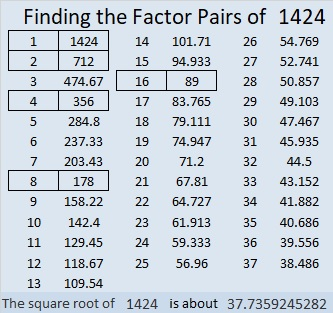1424 is the sum of two squares:
32² + 20² = 1424

1424 is the hypotenuse of a Pythagorean triple:
624-1280-1424 calculated from 32² – 20², 2(32)(20), 32² + 20²

# 1423 Lollipop

Lollipops are candies that easily delight children. Will today’s lollipop-wannabe puzzle be a delight?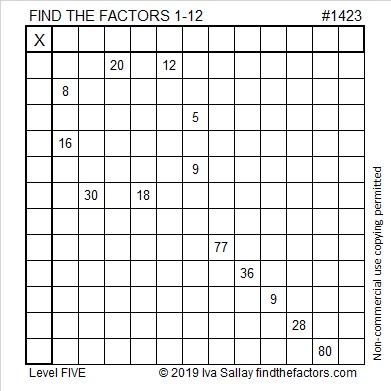Print the puzzles or type the solution in this excel file: 12 Factors 1419-1429

The puzzle number is 1423. Here are a few facts about that number:

• 1423 is a prime number.
• Prime factorization: 1423 is prime.
• 1423 has no exponents greater than 1 in its prime factorization, so √1423 cannot be simplified.
• The exponent in the prime factorization is 1. Adding one to that exponent we get (1 + 1) = 2. Therefore 1423 has exactly 2 factors.
• The factors of 1423 are outlined with their factor pair partners in the graphic below.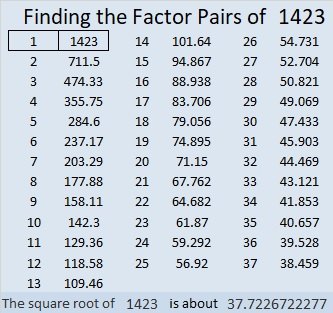How do we know that 1423 is a prime number? If 1423 were not a prime number, then it would be divisible by at least one prime number less than or equal to √1423. Since 1423 cannot be divided evenly by 2, 3, 5, 7, 11, 13, 17, 19, 23, 29 31, or 37, we know that 1423 is a prime number.

# 1422 Candy Corn Mystery

You can begin this candy corn puzzle easily enough, but the logic needed to solve it is a bit complicated. Good luck with this one!Print the puzzles or type the solution in this excel file: 12 Factors 1419-1429

Now I’ll tell you some facts about the puzzle number, 1422:

• 1422 is a composite number.
• Prime factorization: 1422 = 2 × 3 × 3 × 79, which can be written 1422 = 2 × 3² × 79
• 1422 has at least one exponent greater than 1 in its prime factorization so √1422 can be simplified. Taking the factor pair from the factor pair table below with the largest square number factor, we get √1422 = (√9)(√158) = 3√158
• The exponents in the prime factorization are 1, 2, and 1. Adding one to each exponent and multiplying we get (1 + 1)(2 + 1)(1 + 1) = 2 × 3 × 2 = 12. Therefore 1422 has exactly 12 factors.
• The factors of 1422 are outlined with their factor pair partners in the graphic below.1422 is palindrome 1K1 in BASE 29 (K is 20 base 10)
because 1(29²) + 20(29¹) + 1(29º) = 1422.

# 1421 Square-shaped Sweet

This square-shaped puzzle is a Level 3 because you can start with clue 44 and work down the puzzle cell by cell to find the solution. You won’t get into a rhythm with this one, but logic will still help you to find the factors of the clues in order from top to bottom. That’s pretty sweet!Print the puzzles or type the solution in this excel file: 12 Factors 1419-1429

Now here are some sweet facts about the puzzle number 1421:

• 1421 is a composite number.
• Prime factorization: 1421 = 7 × 7 × 29, which can be written 1421 = 7² × 29
• 1421 has at least one exponent greater than 1 in its prime factorization so √1421 can be simplified. Taking the factor pair from the factor pair table below with the largest square number factor, we get √1421 = (√49)(√29) = 7√29
• The exponents in the prime factorization are 2 and 1. Adding one to each exponent and multiplying we get (2 + 1)(1 + 1) = 3 × 2 = 6. Therefore 1421 has exactly 6 factors.
• The factors of 1421 are outlined with their factor pair partners in the graphic below.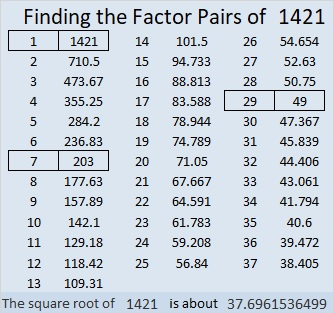1421 is also the hypotenuse of a Pythagorean triple:
980-1029-1421 which is (20-21-29) times 49.

# 1420 Eight-legged Creature

When you look at today’s puzzle, you might see a snowflake or perhaps an eight-legged creature. I hope you will also see lots of common factors that will help you solve this quick puzzle.Print the puzzles or type the solution in this excel file: 12 Factors 1419-1429

Here are some facts about the puzzle number, 1420:

• 1420 is a composite number.
• Prime factorization: 1420 = 2 × 2 × 5 × 71, which can be written 1420 = 2² × 5 × 71
• 1420 has at least one exponent greater than 1 in its prime factorization so √1420 can be simplified. Taking the factor pair from the factor pair table below with the largest square number factor, we get √1420 = (√4)(√355) = 2√355
• The exponents in the prime factorization are 2, 1, and 1. Adding one to each exponent and multiplying we get (2 + 1)(1 + 1)(1 + 1) = 3 × 2 × 2 = 12. Therefore 1420 has exactly 12 factors.
• The factors of 1420 are outlined with their factor pair partners in the graphic below.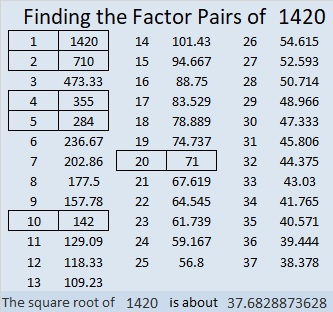1420 is the hypotenuse of a Pythagorean triple:
852-1136-1420 which is (3-4-5) times 284.